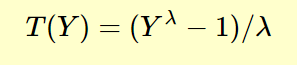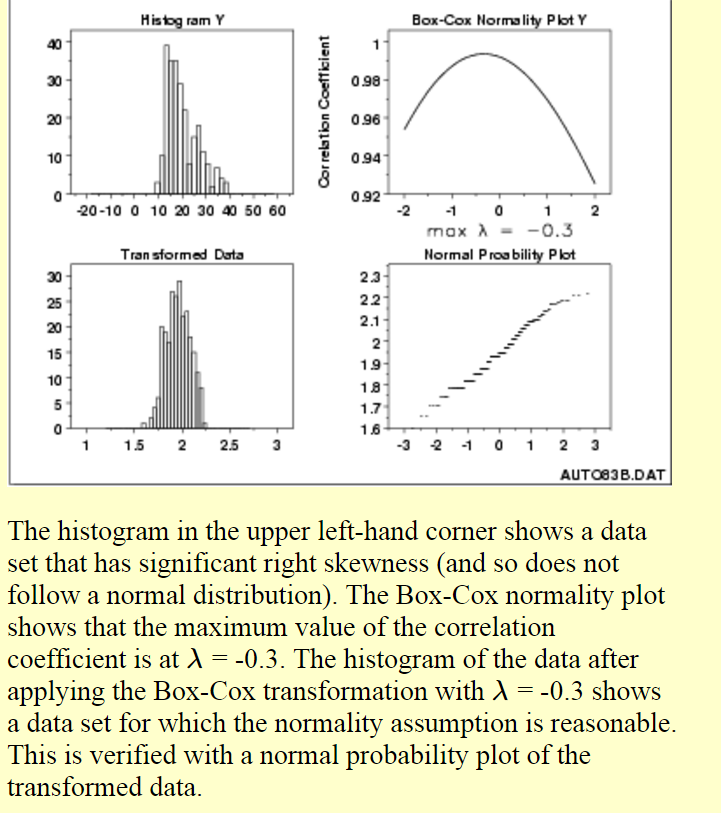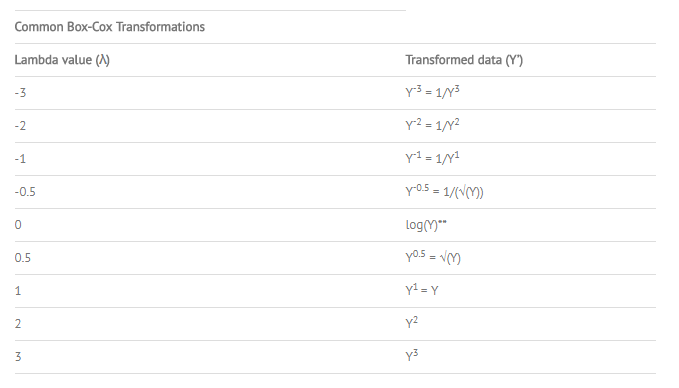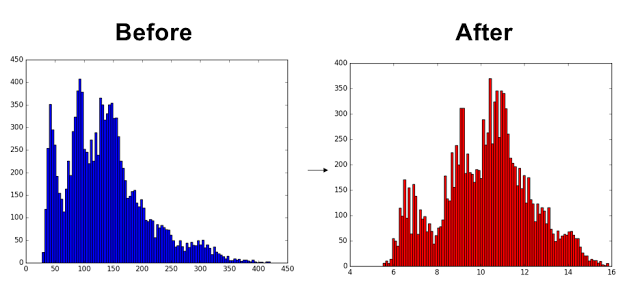Comment on page

# Distribution Transformation

Top 3 methods for handling skewed data. Log, square root, box cox transformations

### BOX COX

(What is the Box-Cox Power Transformation?)
• a procedure to identify an appropriate exponent (Lambda = l) to use to transform data into a “normal shape.”
• The Lambda value indicates the power to which all data should be raised.• Many statistical tests and intervals are based on the assumption of normality.
• The assumption of normality often leads to tests that are simple, mathematically tractable, and powerful compared to tests that do not make the normality assumption.
• Unfortunately, many real data sets are in fact not approximately normal.
• However, an appropriate transformation of a data set can often yield a data set that does follow approximately a normal distribution.
• This increases the applicability and usefulness of statistical techniques based on the normality assumption.IMPORTANT:!! After a transformation (c), we need to measure the normality of the resulting transformation (d) .
• One measure is to compute the correlation coefficient of a normal probability plot => (d).
• The correlation is computed between the vertical and horizontal axis variables of the probability plot and is a convenient measure of the linearity of the probability plot
• In other words: the more linear the probability plot, the better a normal distribution fits the data!
GUARANTEED NORMALITY?
• NO!
• This is because it actually does not really check for normality;
• the method checks for the smallest standard deviation.
• The assumption is that among all transformations with Lambda values between -5 and +5, transformed data has the highest likelihood – but not a guarantee – to be normally distributed when standard deviation is the smallest.
• it is absolutely necessary to always check the transformed data for normality using a probability plot. (d)
+ Additionally, the Box-Cox Power transformation only works if all the data is positive and greater than 0.
+ achieved easily by adding a constant ‘c’ to all data such that it all becomes positive before it is transformed. The transformation equation is then:Finally: An awesome tutorial (dead), here is a new one in python with code examples, there is also another code example here “Simply pass a 1-D array into the function and it will return the Box-Cox transformed array and the optimal value for lambda. You can also specify a number, alpha, which calculates the confidence interval for that value. (For example, alpha = 0.05 gives the 95% confidence interval).”* Maybe there is a slight problem in the python vs R code, details here, but needs investigating.

### MANN-WHITNEY U TEST

(what is?) - the Mann–Whitney U test is a nonparametric test of the null hypothesis that it is equally likely that a randomly selected value from one sample will be less than or greater than a randomly selected value from a second sample.
In other words: This test can be used to determine whether two independent samples were selected from populations having the same distribution.
Unlike the t-test it does not require the assumption of normal distributions. It is nearly as efficient as the t-test on normal distributions.

### NULL HYPOTHESIS

1. 2.
Analytics vidhya
1. 3.
Anova analysis of variance, one way, two way, manova
1. 1.
if the means of two or more groups are significantly different from each other. ANOVA checks the impact of one or more factors by comparing the means of different samples.
2. 2.
A one-way ANOVA tells us that at least two groups are different from each other. But it won’t tell us which groups are different.
3. 3.
For such cases, when the outcome or dependent variable (in our case the test scores) is affected by two independent variables/factors we use a slightly modified technique called two-way ANOVA.
2. 3.
multivariate case and the technique we will use to solve it is known as MANOVA.